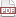### Testing the model using the model.

10 February 10. [link]PDF version

###### Four people are stranded on a desert island

And all they have to eat is a case of canned pears. The joke is that they're all researchers.

The physicist says: we can mill down these coconut husks into lenses, then focus the heat of the sun on the cans. When their temperature rises enough, the seams will burst!'

The chemists says: No, that'll take too long. Instead, we can refine sea water into a corrosive, that will eventually just melt the can open!'

The biologist cuts him off: I don't want salty pears! But I've found a yeast that is capable of digesting metals. With care and cultivation, we can get them to eat the cans open.'

The economist finally stands up and smiles: You are all trying to hard, because it's very simple: assume a can opener.'

Pause for laughter.

I've found that this joke is so commonly told among economists that you can just tell an economist `you're assuming a can opener' and they'll know what you mean. It's also a good joke for parties because people always come up with new ways to open the can. What would the lit major do?

###### Cracking open a model with no tools

Now back to the real world. You are running the numbers on a model regarding data you have collected. To keep this simple, let's say that you're running an Ordinary Least Squares regression on a data set of canned pear sales and education levels. You have the data set, then run OLS to produce a set of coefficients, $\beta$, and $p$-values indicating the odds that the $\beta$s are different from zero.

Those $p$-values are generated using exactly the procedure listed above: assume a distribution of the $\beta$s, write down its CDF, then measure how much of the CDF you assumed lies between zero and $\beta$.

We're still assuming a can opener. We used the assumptions of the model—that errors are normally distributed with mean zero and a variance that is a function of the data—to state the confidence with which we believe the parameters of the very same model.

To make this as clear as possible: we used the model assumptions to write down a probability function, then used that probability function to parts of test the model. But making an assumption about probabilities does not add information.

Pick up any empirically-oriented journal, and in every paper, this is how the confidence intervals will be reported, by assuming that the model is true with certainty and can be used to objectively state probabilities about its own veracity.

So why doesn't all of academia fall apart?

First, many of the assumptions of these models are rooted in objective fact: given such-and-such a setup, errors really will be Normally distributed. We could formalize this by writing down tests to test the assumptions of the main model, though for our purposes there's no point—they'll just fall victim to the same eating-your-own-tail problem. Even lacking extensive testing, if the data generation process is within spitting distance of a Central Limit Theorem, we'll give it benefit of the doubt that there is an objective truth to the distribution.

Second, we can generalize that point to say that the typical competently-written journal article's assumptions are usually pretty plausible, or at least do little harm. When they report that one option is more likely than another, that is often later verified to actually be true, though the authors had used subjective tools to state subjective odds.

Third, we shouldn't believe $p$-values—or any one research study—anyway. A model with fabulous $p$-values will increase our subjective confidence that something real is going on. But if you read that a $p$-value is 99.98%, do you really believe that if you re-gather the data set 10,000 times, the difference will not be significant in exactly two out of 10,000 attempts? Probably not: you just get a sense of greater confidence.

So this works because we treat the process as subjective. The authors made up a model, and used that model to state the odds with which the model is true. But if we agree that the model seems likely, and if we accept that the output odds are just inputs to our own subjective beliefs, then we're doing OK. Problems only arise when we pretend that those $p$-values are derived from some sort of objective probability distribution rather than the author's beliefs as formalized by the model.